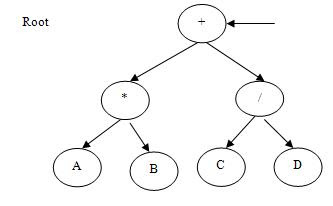# Binary Trees Traversals

Traversal of a binary tree is one of the most important operations required to be done on a binary tree. Traversing of a binary tree is a process of visiting every node in the tree exactly once. Thus it is necessary to visit the nodes of a tree in some linear fashion. There are so many ways in which a binary tree is traversed, but we will consider those techniques in which the left subtree is traversed before the right subtree, such as first visit the node, then traverse left subtree, and finally traverse the right subtree. In such situations where the left subtree is traversed before the right subtree, there are three possible traversal techniques: Inorder, Preorder and Postorder.Figure (a)

## Inorder Traversal

In this, if there is a binary tree ‘T’ then the left subtree of ‘T’ is traversed first, then the root node of ‘T’ is visited and then the right subtree of ‘T’ traversed. Since the left subtree of a binary tree can be itself a binary tree therefore the same procedure is applied to the left subtree. Similalrly the right subtree of a binary tree can be itself a binary tree therefore the same procedure is applied to the right subtree also. Each node of a binary tree is of type BNode and it contains a character as its information.

Here is the function that implements this task.

```Inorder(TNode *rt)
{
if (rt != NULL)
{
Inorder(rt->lchild);
printf("%c\t", rt->data);
Inorder(rt->rchild);
}
}```

If this function is applied on the tree shown in figure-12.38 then the following output would be produced:

A * B + C / D

## Preorder Traversal

In this, if there is a binary tree ‘T’ then the root node of ‘T’ is visited, then the left subtree of ‘T’ is traversed first, and then finally the right subtree of ‘T’ traversed. Since the left subtree of a binary tree can be itself a binary tree therefore the same procedure is applied to the left subtree. Similalrly the right subtree of a binary tree can be itself a binary tree therefore the same procedure is applied to the right subtree also.

Here is the function that implements this task.

```Preorder(TNode *rt)
{
if (rt != NULL)
{
printf("%c\t", rt->data);
Preorder(rt->lchild);
Preorder(rt->rchild);
}
}```

If this function is applied on the tree shown in figure-38 then the following output would be produced:

+ *  A B / C D

## Postorder Traversal

In this, if there is a binary tree ‘T’ then the right subtree of ‘T’ traversed first, then the left subtree of ‘T’ is traversed first, and then finally then the root node of ‘T’ is visited. Since the left subtree of a binary tree can be itself a binary tree therefore the same procedure is applied to the left subtree. Similarly the right subtree of a binary tree can be itself a binary tree therefore the same procedure is applied to the right subtree also.

Here is the function that implements this task.

```Postorder(TNode *rt)
{
if (rt != NULL)
{
Postorder(rt->lchild);
Postorder(rt->rchild);
printf("%c\t", rt->data);
}
}```

If this function is applied on the tree shown in figure-38 then the following output would be produced:

A  B * C D /  +